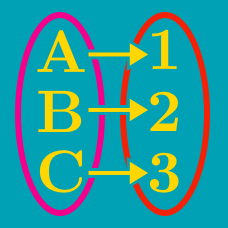Probability

# Bijection, Injection and Surjection Problem Solving

Suppose that $10$ dice are rolled. Each die is a regular $6$-sided die with numbers $1$ through $6$ labelled on the sides. How many different distinct sums of all 10 numbers are possible?

Details and assumptions

The sums $5 = 1+4$ and $5 = 2 + 3$ are the same sum (namely 5), and should only be counted once.

Let $A=\{1, 2, \ldots, 6\}$ and $B=\{b_1, b_2, \ldots , {b}_{5}\},$ where the $b_i$ are all distinct. How many distinct functions from $A$ to $B$ are possible?

In New York city, all the streets are arranged in a grid. A hospital is located 5 blocks east and 6 blocks north of an accident. How many ways are there to get there, if we only go 1 block north or 1 block east at each intersection?

A product day is a day where the numerical value of the date, multiplied by the numerical value of the month, is equal to the numerical value of the year.

Starting from 1st of January, 1AD, how many product days are there?

Details and assumptions

Assume that the current convention of days and months extend back to 1st of January, 1 AD. You should include the 1st of January, 1AD into your count.

25th of December, 300 AD is a product day, because $25 \times 12 = 300$.

Mary has enrolled in $6$ courses: Chemistry, Physics, Math, English, French and Biology. She has one textbook for each course and wants to place them on a shelf. How many ways can she arrange the textbooks so that the English textbook is placed before the French textbook?

Details and assumptions

If the books are ordered from left to right, then the "English textbook is placed before the French textbook" means that the English textbook was placed to the left of the French textbook. They do not need to be immediately beside each other.

×